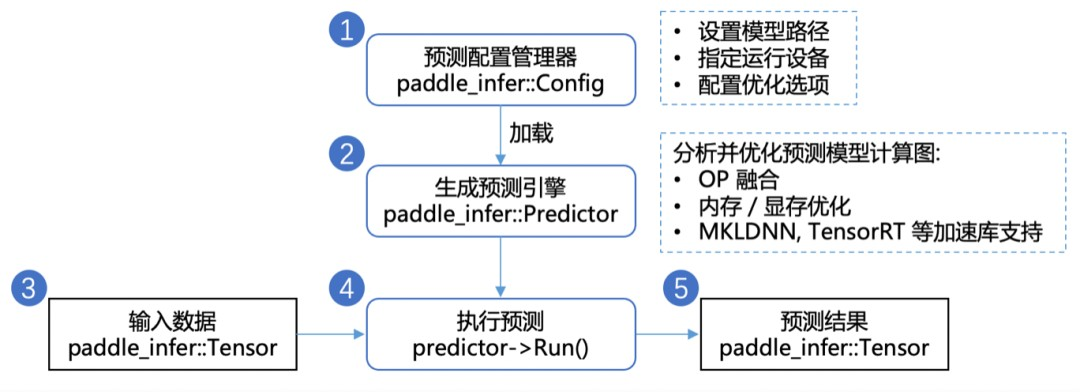# 1、方案介绍

## 数据集介绍

``````{
"id": 98,
"text": "黄帝道：什麽叫重实？岐伯说：所谓重实，如大热病人，邪气甚热，而脉象又盛满，内外俱实，便叫重实",
"annotations": [
{
"Q": "重实是指什么？",
"A": "所谓重实，如大热病人，邪气甚热，而脉象又盛满，内外俱实，便叫重实"
},
{
"Q": "重实之人的脉象是什么样？",
"A": "脉象又盛满"
}
],
"source": "黄帝内经翻译版"
}
``````

## 技术点介绍

• 构建中医 MRC 数据集，使用 PaddleNLP 搭建、训练并调优阅读理解模型；
• 采用多种内置模型进行实验，最终确定使用 Roberta 阅读理解模型；

### RoBERTa 阅读理解模型

• 训练时间更长，batch size 更大，训练数据更多；

• 移除了 next predict loss；

• 训练序列更长；

### 最终效果呈现# 02 设计流程展示

## 环境配置

https://aistudio.baidu.com/aistudio/projectdetail/5166465

## 方案设计## 数据处理

• lid: 段落 id

• ltext: 段落文本

• lannotations: 每个段落拥有一个 annotations，其中包含1~4对（问题、答案）

• lＱ：问题

• lＡ：答案

``````
{
'id': 'xx', 'title': 'xxx',
'context': 'xxxx',
'question': 'xxxxx',
}
``````

# 模型训练与策略选择

## 设置 Fine-tune 优化策略

``````
# 参数配置

# 训练过程中的最大学习率
learning_rate = 3e-5
# 训练轮次
epochs = 2
# 学习率预热比例
warmup_proportion = 0.1
# 权重衰减系数，类似模型正则项策略，避免模型过拟合
weight_decay = 0.01

lr_scheduler = ppnlp.transformers.LinearDecayWithWarmup(learning_rate, num_training_steps, warmup_proportion)

# Generate parameter names needed to perform weight decay.
# All bias and LayerNorm parameters are excluded.
decay_params = [
p.name for n, p in model.named_parameters()
if not any(nd in n for nd in ["bias", "norm"])
]
learning_rate=lr_scheduler,
parameters=model.parameters(),
weight_decay=weight_decay,
apply_decay_param_fun=lambda x: x in decay_params)
``````

## 设计损失函数

``````
def init (self):
def  forward(self,  y,  label):
start_logits,  end_logits  =  y    # both shape are [batch_size, seq_len]
start_position, end_position = label
logits=start_logits,  label=start_position,  soft_label=False) start_loss = paddle.mean(start_loss)
end_loss = paddle.nn.functional.softmax_with_cross_entropy( logits=end_logits,  label=end_position,  soft_label=False)

loss = (start_loss + end_loss) / 2 return loss
``````

## 模型训练

• 从 dataloader 中取出一个 batch data；

• 将 batch data 喂给 model，做前向计算；

• 将前向计算结果传给损失函数，计算 loss；

• loss 反向回传，更新梯度。重复以上步骤。

# 03 预测部署

## 使用推理库预测• 将模型拷贝到本地，并按照接口要求封装好方法；
``````
``````
• 加载用户输入的 context 和 question，并利用模型返回 answer；